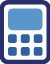## Saturday, October 24, 2009

### A basic probability question concerning throwing a dieIf a fair sided die is thrown 6x, what is the probability of it landing 3x on the number 6?

[BTW, C(n,k) refers to combination. While x^y refers to exponentation.]

Suppose you number the throws 1 to 6:
C(6,3) => which of the 6 throws hits a 6. (There should be only exactly 3. That's why C(6,3).

Then you have to figure out the number of ways the other 3 non-6 throws could come out:
=> 5^3
since they can be anything from 1 to 5.

Multiply those 2 things to get the total number of ways to throw 3 sixes with 6 throws.

But that doesn't answer the problem completely. To get the probability, you need to divide that by the total number of ways to throw a 6 sided die, which is 6^6.

So the complete answer is: (C(6,3)*5^3) / 6^6

So this might be useful for someone preparing for one of the acronym standardized tests like the GRE or the GMAT. :)